# Borsuk problem

(diff) ← Older revision | Latest revision (diff) | Newer revision → (diff)
One of the fundamental problems in combinatorial geometry: Is it possible, for any bounded set of diameter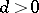in an-dimensional Euclidean space, to make a decomposition into not more thansubsets with diameters smaller than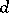? The problem was formulated by K. Borsuk  who noted that it was not possible to subdivide an-dimensional simplex and an-dimensional sphere inintoparts of a smaller diameter. The problem has a positive solution for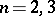, but only partial results have been obtained for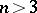. Thus, for instance, the problem has been positively solved for any bounded smooth convex set in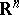. It has been proved that the solution of Borsuk's problem can be reduced to the case of sets of constant width. Ifis the smallest number of parts of a diameter smaller thaninto which a set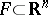can be subdivided, then the equality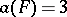is valid for a figure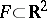of diameterif and only if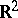contains a unique figure of constant widthcontaining. This fact cannot be directly generalized to the case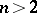. The Borsuk problem is closely related to the illumination problem and to the Hadwiger hypothesis, which is a generalization of the Borsuk problem in whichis replaced by a finite-dimensional normed space.